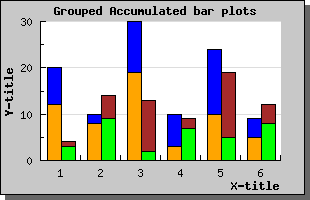Contents Previous Next

### 8.2.7 Using grouped accumulated bar graphs

It is perfectly possible to combine the previous bar types to have grouped accumulated bar plots. This is done by just adding the different accumulated plots to a group bar plot, for example the following code would do that.

```   // Create all the 4 bar plots \$b1plot  = new BarPlot(\$data1y); \$b1plot->SetFillColor( "orange"); \$b2plot  = new BarPlot(\$data2y); \$b2plot->SetFillColor( "blue"); \$b3plot  = new BarPlot(\$data3y); \$b3plot->SetFillColor( "green"); \$b4plot  = new BarPlot(\$data4y); \$b4plot->SetFillColor( "brown"); // Create the accumulated bar plots \$ab1plot  = new AccBarPlot (array(\$b1plot ,\$b2plot)); \$ab2plot  = new AccBarPlot (array(\$b3plot ,\$b4plot)); // Create the grouped bar plot \$gbplot  = new GroupBarPlot (array(\$ab1plot ,\$ab2plot)); // ...and add it to the graph \$graph->Add( \$gbplot); ```

Putting this together in an example would then produce the graph as shown belowFigure 39: Combining grouped and accumulated bar plots [src]

Contents Previous Next